MORE IN Signals & Systems
Total marks: --
Total time: --
INSTRUCTIONS
(1) Assume appropriate data and state your reasons
(2) Marks are given to the right of every question
(3) Draw neat diagrams wherever necessary

1 (a) Define signal and system with example. And briefly explain operations performed on independent variable of the signal.
6 M
1 (b) Determine whether the following signal is energy signal or power signal and calculate its energy of power $x(t)=rect \left ( \dfrac {t}{t_o} \right ) \cos \omega_o t.$
4 M
1 (c) Find the whether the following system is stable, memory less, linear and time invariant? y(t)=sin[x(t+2)]
4 M
1 (d) Two signals x(t) and g(t) as shown in Fig Q1(d). Express the signals x(t) in terms of g(t).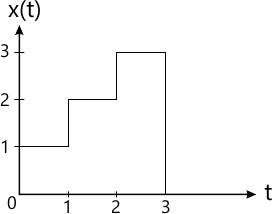Fig.1 d (i)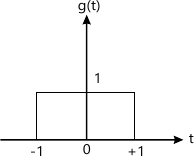Fig. 1 d (ii)

6 M

2 (a) Given the signal x(t) as shown in Fig Q2(a). Sketch the following: $i) \ x(-2t+3) \\ ii) \ x\left ( \dfrac {1}{2}-2 \right )$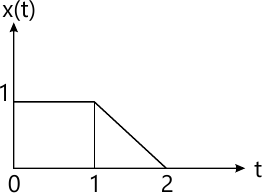4 M
2 (b) For a DT LTI system to be stable show that $S\overset {\Delta}= \sum^{k=+\infty}_{k=-\infty} |h(K)|<\infty$
5 M
2 (c) Two discrete time LTI systems are connected in cascade as shown in Fig Q2(c). Determine the unit sample response of this cascade connection.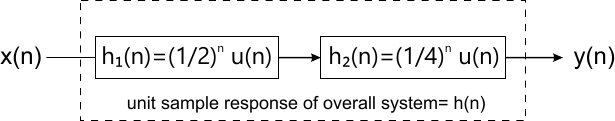6 M
2 (d) Find convolution of 2 finite duration sequences,
h(n)=anu(n) for all n and
x(n)=bnu(n) for all n
i) when a?b
ii) when a=b
5 M

3 (a) Determine the LTI system characterized by impulse response. $i) \ h(n)=n\left ( \dfrac {1}{2} \right )^n u(n) \\ ii) h(t)=e^{-t}u(t+100)$ Stable and causal.
6 M
3 (b) Find the forced response of the following system; $y(n)- \dfrac {1}{4}y(n-1)- \dfrac {1}{8} y(n-2)= x(n)+x(n-1) \ for \ x(n)= \left ( \dfrac {1}{8} \right )^n u(n)$
8 M
3 (c) Draw direct form II implementation for the system described by the following equation and indicate number of delay elements, adders, multipliers.
y(n)-0.25y(n-1)-0.125y(n-2)-x(n)-x(n-2)=0
6 M

4 (a) Prove the following properties of DTFS:
i) Convolution in time ii) Modulation theorem
6 M
4 (b) Determine the complex exponential Fourier series for periodic rectangular pulse train shown in Fig Q4(b). Plot its magnitude and phase spectrum.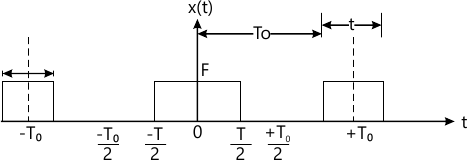8 M
4 (c) Determine the DTFS representation for the signal $x(n)=\cos \left ( \dfrac {n\pi}{3}$ Plot the spectrum of x(n).
6 M

5 (a) State and prove the following properties of DTFT:
(i) Parseval's theorem ii) Linearity
6 M
5 (b) Find the DTFT on the signals shown, $i) \ x(n) = \left ( \dfrac {1}{4} \right )^n u(n+4) \\ ii) x(n)= u(n)$
8 M
5 (c) Find the inverse Fourier transform of the rectangular shown below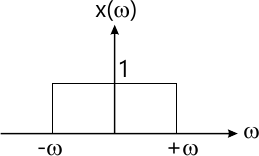6 M

6 (a) Consider the continuous time LTI system described by, $\dfrac {d}{dt} y(t)+2y(t)=x(t)$ Using FT, find the output y(t) to each of the following input signals.
i) x(t)=e-tu(t)
ii) x(t)=u(t)
8 M
6 (b) Find the Nyquist rate and Nyquist interval for each of the following signals:
i) x(t)=sin c2 (200t)
ii) x(t)=2 sin c(50t) sin(5000?t)
6 M
6 (c) An LTI system is described by $H(f)=\dfrac {4} {2+j2\pi f}$ find its response y(t) if the input is x(t)=u(t).
6 M

7 (a) Define ROC and list its properties.
4 M
7 (b) State and prove time reversal property of z-transform.
4 M
7 (c) Determine the inverse z-transform of $x(z)=\dfrac {1}{(1+z^{-1})(1-z^{-1})^2} ; ROC;|z|>1$
6 M
7 (d) Determine z-transform and ROC of $x(n)= \left ( \dfrac {1}{3} \right )^n \sin \left ( \dfrac {\pi}{4}n \right )u(n)$
6 M

8 (a) A causal, stable discrete time system is defined by $y(n)= \dfrac {5}{6} y(n-1)-\dfrac {1}{6}y(n-2)+x(n)-2x(n-1) Determine : i) System function H(z) and magnitude response at zero frequency ii) Impulse response of the system. iii) Output y(n) for x(n)=?(n)- 1/3 ?(n-1) 12 M 8 (b) Solve the following difference equation for the given initial conditions and input, \[ y(n)-\dfrac {1}{9} y(n-2)=x(n-1) \\ with \ y(-1)=0, y(-2)=1 \ and \ x(n)=3u(n)$
8 M

More question papers from Signals & Systems